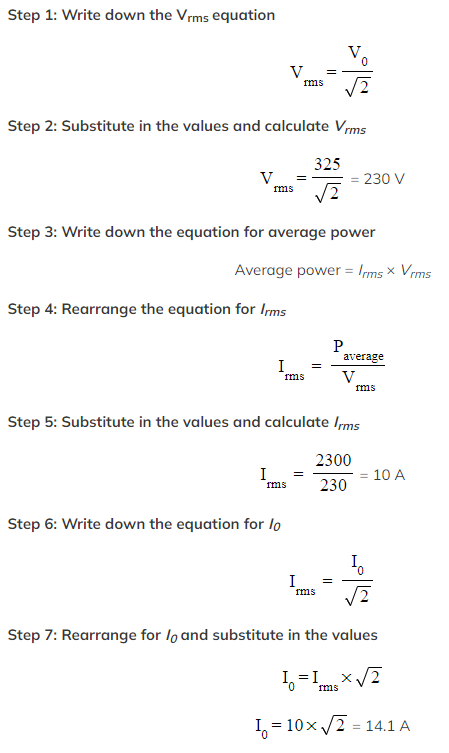# IB DP Physics: HL复习笔记11.2.2 Root-Mean-Square Current & Voltage

### Root-Mean-Square Current & Voltage

• Direct current sources provide a constant voltage and current over time, making it easy to measure
• In situations involving alternating voltage and current, the average values of voltage and current will always be zero
• This can make it difficult to measure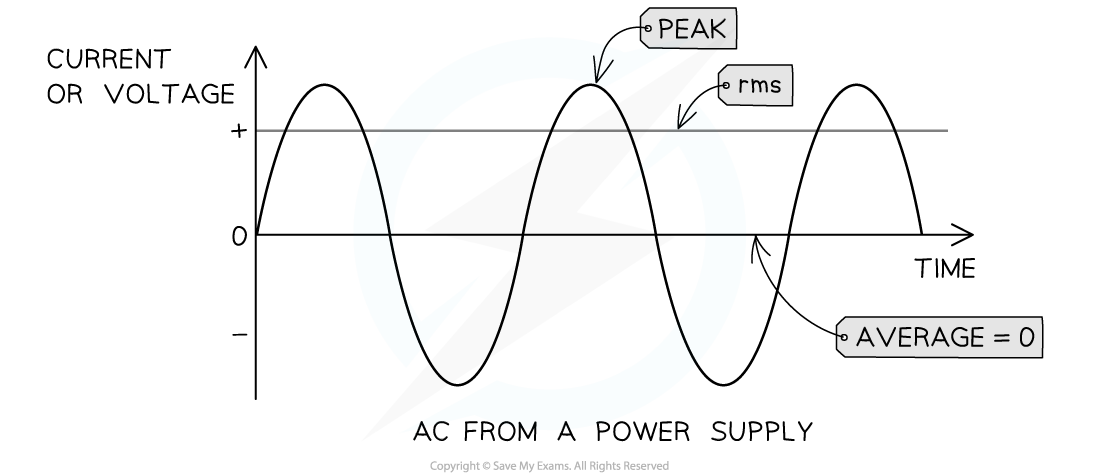The mean value for alternating current and voltage is always zero

• The use of root mean square values gets around this problem
• First remove all the negative signs by simply squaring the peak current, or voltage
• Find the average of the squared value
• And finally, take the square root
• Root-mean-square (rms) values of current, or voltage, are a useful way of comparing a.c current, or voltage, to its equivalent direct current (d.c), or voltage
• The rms values represent the direct current, or voltage, values that will produce the same heating effect, or power dissipation, as the alternating current, or voltage
• The rms value of an alternating current is defined as:

The square root of the mean of squares of all the values of the current in one cycle

• An alternate definition is:

The equivalent direct current that produces the same power

•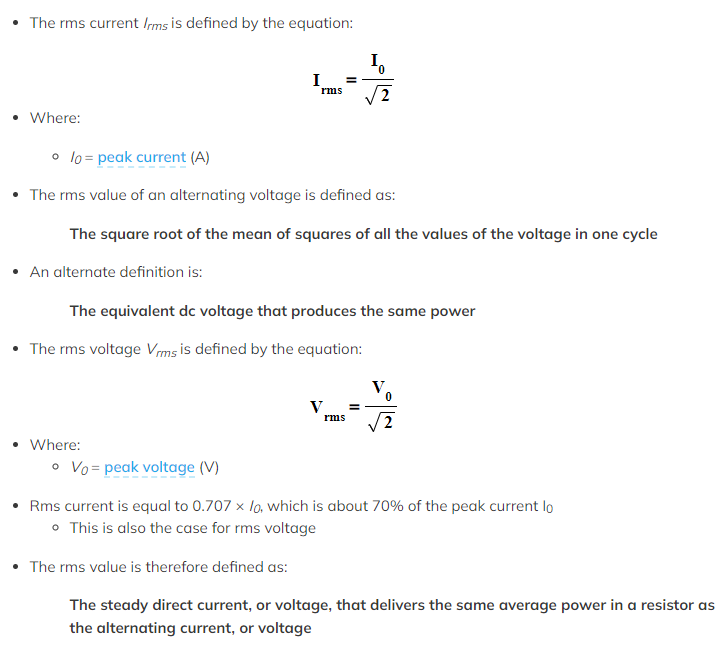A resistive load is any electrical component with resistance eg. a lamp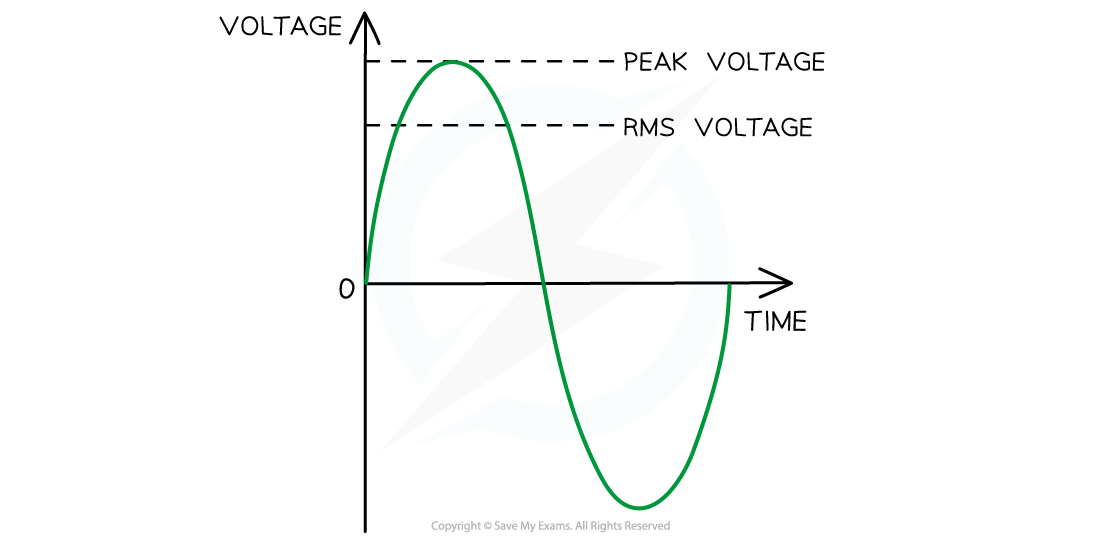Vrms and peak voltage. The rms voltage is about 70% of the peak voltage

#### Worked Example

An electric oven is connected to a 230 V root mean square (rms) mains supply using a cable of negligible resistance.

Calculate the peak-to-peak voltage of the mains supply.

Step 1: Write down the Vrms equation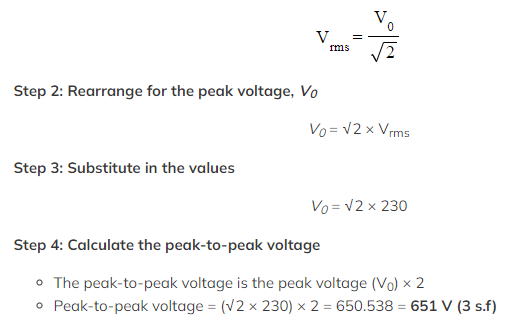#### Exam Tip

Remember to double-check the units on the alternating current and voltage graphs. These are often shown in milliseconds (ms) instead of seconds (s) on the x-axis.

### Average Power Calculations

• The average power of a supply is the product of the rms current and voltage:

Average power = Irms × Vrms

#### Worked Example

What is the maximum current supplied to a 2300 W kettle which is connected to an a.c. supply of peak voltage 325 V?